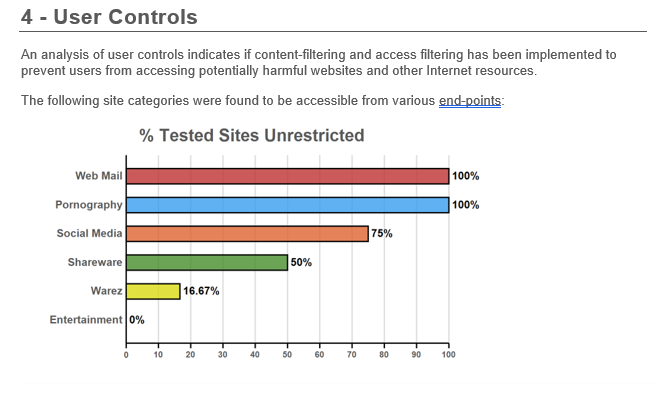## Outbound Security Report - User Controls explained

The User Controls Section of the Out Security Report contains a graph showing the percentage of sites that are unrestricted based on the number of machines sampled.

Here is the formula being used to calcuate those percentages. (Unrestricted Sites tested  X  Number of Machines sampled divided by 100)  X  (Number of Sites determined unrestricted)

Let's take the Warez Category in example below and plug the numbers into the formula.

(Unrestricted Sites under Warez (2)  X  Number of Machines sampled (3) divided by 100) x (Number of Sites determined unrestricted)
2 x 3 / 100 = 16.67 x 1= 16.67%